# This section is a collection of some useful things to know that may help to become more productive following an introduction to R

### Data import

http://www.metoffice.gov.uk/climate/uk/stationdata/

The data for Hurn, which is the station closest to Bournemouth can be seen here.

http://www.metoffice.gov.uk/climate/uk/stationdata/hurndata.txt

These are tab delimited text files. They should be quite easy to import into R but they still have some commonly found issues.

### Skipping lines

The first thing we might notice is that the data begin with a few lines of meta data. If we use read.table to read in the lines we would need to skip these. There are also two header lines, one giving the type of measurement and one providing details on the units.

``````datasource <- "http://www.metoffice.gov.uk/climate/uk/stationdata/hurndata.txt"
d <- read.table(datasource, skip = 7, fill = T, col.names = c("Yr",
"Mon", "tmax", "tmin", "af", "rain", "sun", "comments"))
``````
``````##     Yr Mon tmax tmin af  rain sun comments
## 1 1957   1  9.1  2.5  7  73.0 ---       NA
## 2 1957   2  9.6  2.7  8 100.5 ---       NA
## 3 1957   3 12.9  5.5  4  61.3 ---       NA
## 4 1957   4 14.2  4.4  2   5.5 ---       NA
## 5 1957   5 16.1  5.5  1  43.7 ---       NA
## 6 1957   6 22.2  8.8  0  26.4 ---       NA
``````

### Removing unwanted characters

One of the commonest problems is caused by the addition of characters in a vector that should be numeric. This causes the whole column to be coerced to a factor.

The gsub function in R is much more powerful than find and replace in Excel as it can use regular expressions. These are a standard feature of programming languages that allow patterns to be defined. The following useful little function will replace all characters that are not either numbers (0-9) or a decimal point (.) with blanks and coerce the result back to a number.

``````clean <- function(x) {
x <- as.character(x)
x <- gsub("[^0-9,/.]", "", x)
as.numeric(x)
}
``````

Testing.

``````x <- "##%Meas126.34cm"
clean(x)
``````
``````##  126.3
``````

You may still have problems if numbers or decimal points are used in what are meant to be comments or unit definitions. For example this might not work quite as expected because the 2 will not be removed.

``````x <- "123.4cm^2"
clean(x)
``````
``````##  123.4
``````

However it is clearly difficult to cater for all eventualities. When reading in files with non standard formats it is usually necessary to “eyeball” the original files first in order to see what they contain.

We can now clean each column.

``````d\$Mon <- clean(d\$Mon)
cleandf <- function(x) {
x <- data.frame(lapply(x, clean))
x
}
d <- cleandf(d)

``````

Now we can try some anayses.

``````library(car)
``````
``````## Loading required package: nnet
``````
``````## Attaching package: 'car'
``````
``````## The following object(s) are masked from 'package:gtools':
##
## logit
``````
``````library(gplots)
Boxplot(rain ~ Mon, labels = Yr, data = d, col = "grey")
``````
``````##  "2000" "2012" "1971" "1960" "1966"
``````
``````plotmeans(rain ~ Mon, connect = F, add = T, data = d, n.label = F,
barcol = "red", col = "red", pch = 21)
``````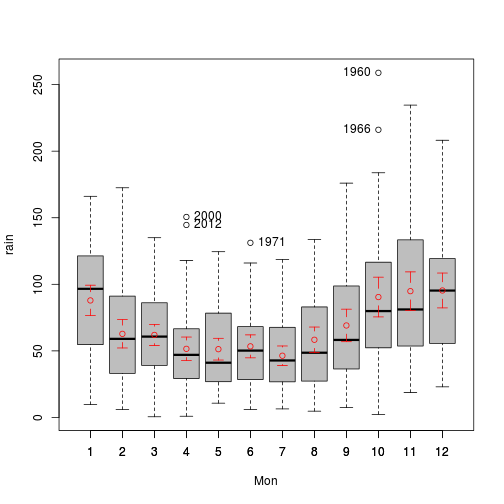### Adding a spline with confidence intervals.

Sourcing in this file http://tinyurl.com/Rexample/IntroFunctions.R will provide you with the simple ablines2 function that I have written. This will add confidence intervals to a plot using the standard errors provided by the predict method when models do not provide confidence intervals. You need to attach the dataframe first before using it.

``````ablines <- function(mod, type = "confidence", ...) {
a <- names(mod\$model)
b <- paste("newdata <- data.frame(", a, "=seq(min(", a, "),max(", a, "),l=100))",
sep = "")
eval(parse(text = b))
a <- predict(mod, newdata, interval = type)
matlines(newdata[, 1], a, ...)
}

ablines2 <- function(mod, ...) {
a <- names(mod\$model)
b <- paste("newdata <- data.frame(", a, "=seq(min(", a, "),max(", a, "),l=100))",
sep = "")
eval(parse(text = b))
a <- predict(mod, newdata, type = "response", se = TRUE)
a\$upper = a\$fit + a\$se.fit * 2
a\$lower = a\$fit - a\$se.fit * 2
a <- data.frame(a\$fit, a\$upper, a\$lower)
matlines(newdata[, 1], a, ...)
}

``````
``````library(mgcv)
``````
``````## This is mgcv 1.7-18. For overview type 'help("mgcv-package")'.
``````
``````Boxplot(rain ~ Mon, labels = Yr, data = d, col = "grey")
``````
``````##  "2000" "2012" "1971" "1960" "1966"
``````
``````mod <- gam(rain ~ s(Mon), data = d)
attach(d)
ablines2(mod, lwd = 2, col = 2, lty = 2)
``````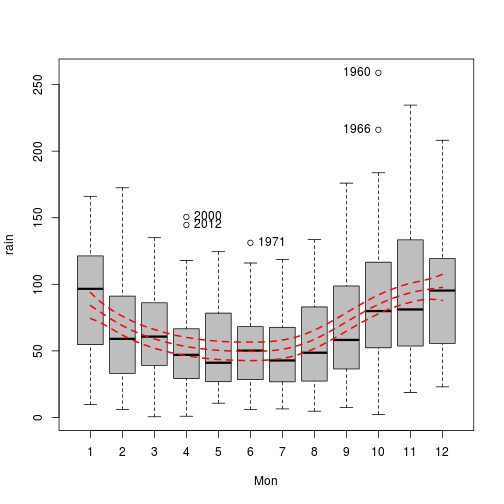``````detach(d)
``````

### More reshaping

Before looking at yearly means we need to remove 2012 as this only has partial data.

``````d <- d[d\$Yr < 2012, ]
``````
``````library(reshape)
``````
``````## Loading required package: plyr
``````
``````## Attaching package: 'reshape'
``````
``````## The following object(s) are masked from 'package:plyr':
##
## rename, round_any
``````
``````d1 <- melt(d, id = c("Yr", "Mon"), meas = c("tmax", "tmin", "rain"))
d2 <- data.frame(cast(d1, Yr ~ variable, mean))
``````
``````##     Yr  tmax  tmin   rain
## 1 1957 14.86 6.150  61.33
## 2 1958 13.87 6.025  77.33
## 3 1959 15.61 6.000  71.26
## 4 1960 14.10 6.167 104.37
## 5 1961 14.81 5.992  62.88
## 6 1962 13.08 5.000  56.19
``````
``````str(d2)
``````
``````## 'data.frame':    55 obs. of  4 variables:
##  \$ Yr  : num  1957 1958 1959 1960 1961 ...
##  \$ tmax: num  14.9 13.9 15.6 14.1 14.8 ...
##  \$ tmin: num  6.15 6.03 6 6.17 5.99 ...
##  \$ rain: num  61.3 77.3 71.3 104.4 62.9 ...
``````

Are wet years colder than dry years?

``````plot(rain ~ tmin, data = d2)
mod <- lm(rain ~ tmin, data = d2)
attach(d2)
ablines(mod)
``````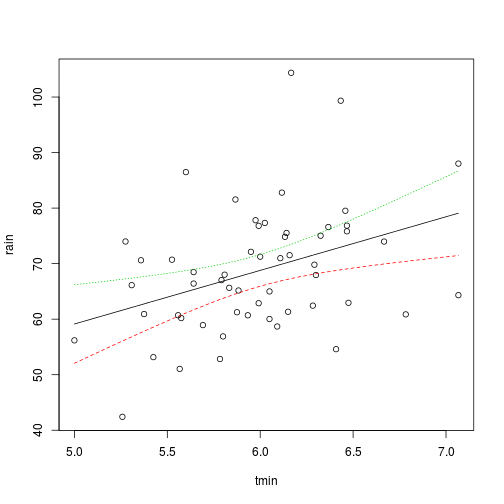``````anova(mod)
``````
``````## Analysis of Variance Table
##
## Response: rain
##           Df Sum Sq Mean Sq F value Pr(>F)
## tmin       1    973     973    8.74 0.0046 **
## Residuals 53   5900     111
## ---
## Signif. codes:  0 '***' 0.001 '**' 0.01 '*' 0.05 '.' 0.1 ' ' 1
``````

It appears that the relationship is the reverse. Cool years are slightly drier on average. What about the mean maximum temperature?

``````plot(rain ~ tmax, data = d2)
mod <- lm(rain ~ tmax, data = d2)
ablines(mod)
``````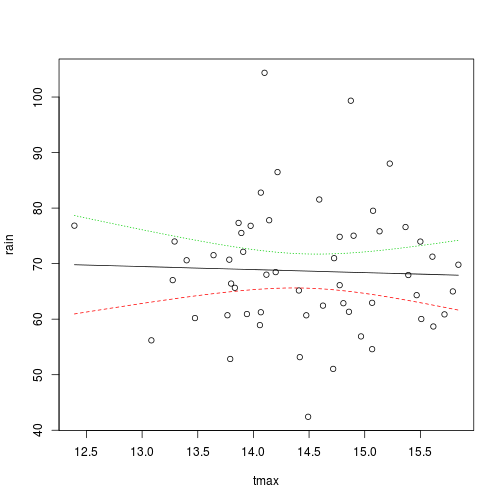``````anova(mod)
``````
``````## Analysis of Variance Table
##
## Response: rain
##           Df Sum Sq Mean Sq F value Pr(>F)
## tmax       1     10     9.7    0.07   0.79
## Residuals 53   6863   129.5
``````

There is no apparent relationship.

Is there any evidence of a trend over time?

``````plot(rain ~ Yr, data = d2)
mod <- lm(rain ~ Yr, data = d2)
ablines(mod)
``````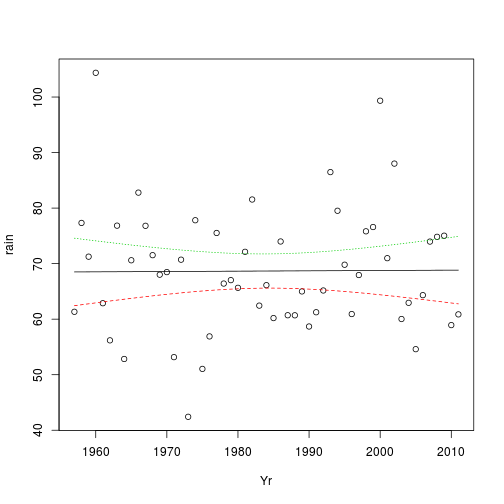``````anova(mod)
``````
``````## Analysis of Variance Table
##
## Response: rain
##           Df Sum Sq Mean Sq F value Pr(>F)
## Yr         1      1     0.5       0   0.95
## Residuals 53   6873   129.7
``````
``````confint(mod)
``````
``````##                 2.5 %   97.5 %
## (Intercept) -328.3771 441.4682
## Yr            -0.1879   0.2001
``````

None for rainfall. What about temperature?

``````plot(tmin ~ Yr, data = d2)
mod <- lm(tmin ~ Yr, data = d2)
ablines(mod)
``````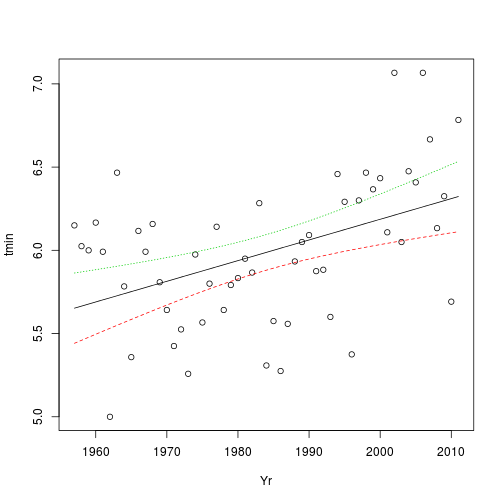``````anova(mod)
``````
``````## Analysis of Variance Table
##
## Response: tmin
##           Df Sum Sq Mean Sq F value  Pr(>F)
## Yr         1   2.14   2.137    13.7 0.00052 ***
## Residuals 53   8.30   0.157
## ---
## Signif. codes:  0 '***' 0.001 '**' 0.01 '*' 0.05 '.' 0.1 ' ' 1
``````
``````confint(mod)
``````
``````##                  2.5 %   97.5 %
## (Intercept) -32.025250 -5.27214
## Yr            0.005676  0.01916
``````
``````plot(tmax ~ Yr, data = d2)
mod <- lm(tmax ~ Yr, data = d2)
ablines(mod)
``````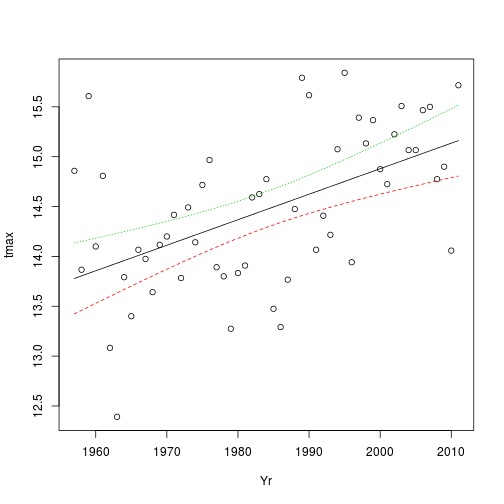``````anova(mod)
``````
``````## Analysis of Variance Table
##
## Response: tmax
##           Df Sum Sq Mean Sq F value  Pr(>F)
## Yr         1   9.08    9.08    20.5 3.4e-05 ***
## Residuals 53  23.51    0.44
## ---
## Signif. codes:  0 '***' 0.001 '**' 0.01 '*' 0.05 '.' 0.1 ' ' 1
``````
``````confint(mod)
``````
``````##                 2.5 %    97.5 %
## (Intercept) -58.82391 -13.80216
## Yr            0.01425   0.03694
``````

A clear trend. However the result may be affected by serial autocorrelation, which should usually be built into time series analysis. Is there any autocorrelation of the residuals?

``````plot(acf(residuals(mod)))
``````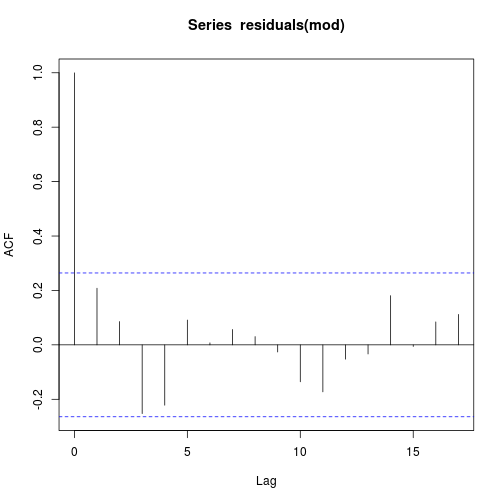``````library(lmtest)
``````
``````## Loading required package: zoo
``````
``````## Attaching package: 'zoo'
``````
``````## The following object(s) are masked from 'package:base':
##
## as.Date, as.Date.numeric
``````
``````dwtest(tmin ~ Yr, data = d2)
``````
``````##
##  Durbin-Watson test
##
## data:  tmin ~ Yr
## DW = 1.782, p-value = 0.1695
## alternative hypothesis: true autocorrelation is greater than 0
##
``````
``````detach(d2)
``````

Running an analysis over several stations

The web site has data for more climate stations. We could write a little function to pull down the data for each.

``````getstatdata <- function(x = "hurn") {
x1 <- paste("http://www.metoffice.gov.uk/climate/uk/stationdata/", x, "data.txt",
sep = "")
d <- read.table(x1, skip = 7, fill = T, col.names = c("Yr", "Mon", "tmax",
d <- cleandf(d)
d <- data.frame(station = x, d)
d
}
``````

Now if we can form a list of the station names we can get data from across the south of England.

``````a <- c("hurn", "southampton", "yeovilton", "chivenor", "camborne",
"oxford", "heathrow", "eastbourne", "manston", "rossonwye", "cardiff", "cambridge")
d <- lapply(a, getstatdata)
``````

The do.call function will rbind the results into a single data frame.

``````d <- do.call("rbind", d)
d <- d[!is.na(d\$rain), ]
d <- d[d\$Yr < 2012, ]
``````

### Applying the same analysis to each station

To analyse each station in turn we could use a loop. However R programmers prefer to use a split and apply approach. So we can roll together some of the previous code to form a little function that models rainfall. Notice that there are a few extra lines to form titles for the plots.

``````rainmodels <- function(d) {
d <- droplevels(d)
nm <- toupper(levels(d\$station))
nm <- paste(nm, " Mean annual rain =", round(mean(tapply(d\$rain, d\$Yr, sum)),
0))

attach(d)
Boxplot(rain ~ Mon, labels = Yr, data = d, col = "grey", main = nm)
mod <- gam(rain ~ s(Mon), data = d)
ablines2(mod, lwd = 2, col = 2, lty = 2)
detach(d)
####################################
d1 <- melt(d, id = c("Yr", "Mon"), meas = c("rain"))
d2 <- data.frame(cast(d1, Yr ~ variable, sum))
attach(d2)
####################################################
mod <- lm(rain ~ Yr)
an <- anova(mod)
pval <- round(an[1, ], 2)
nm <- paste(nm, "\n", "P value=", pval)
plot(rain ~ Yr, pch = 21, bg = 2, main = nm)
ablines(mod)
detach(d2)
}
``````
``````lapply(split(d, d\$station), rainmodels)
``````
``````## Warning: matrix not positive definite
``````
``````## Warning: matrix not positive definite
``````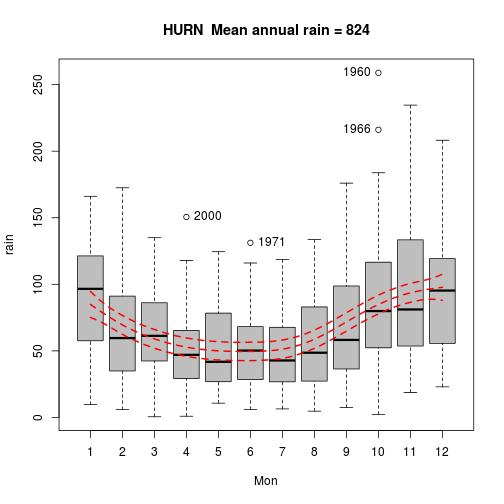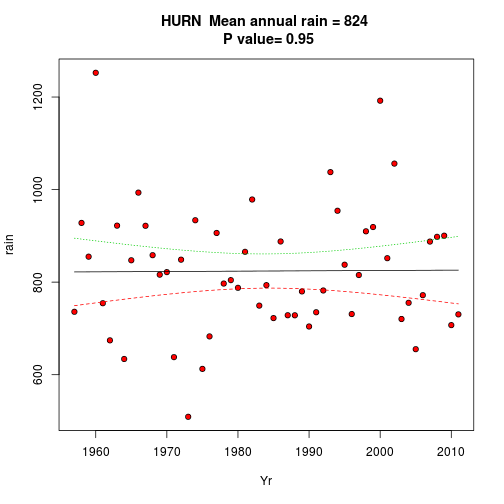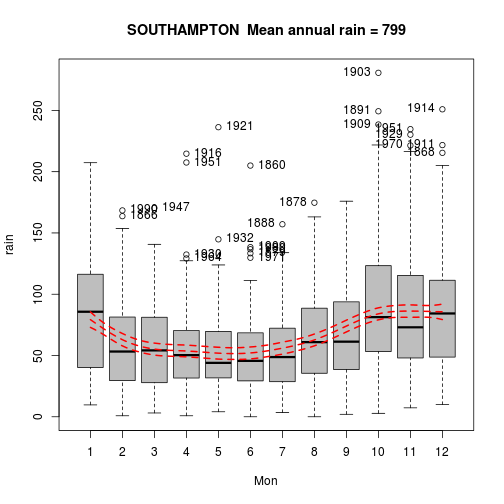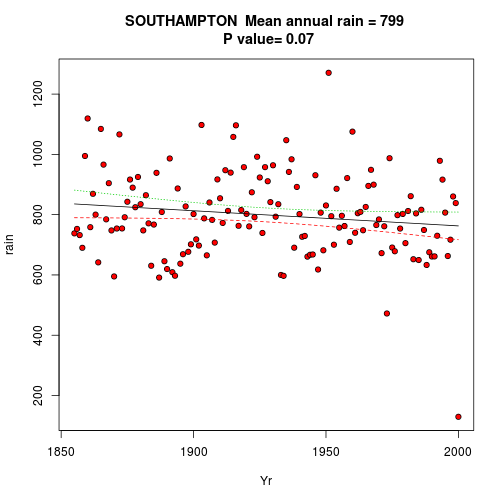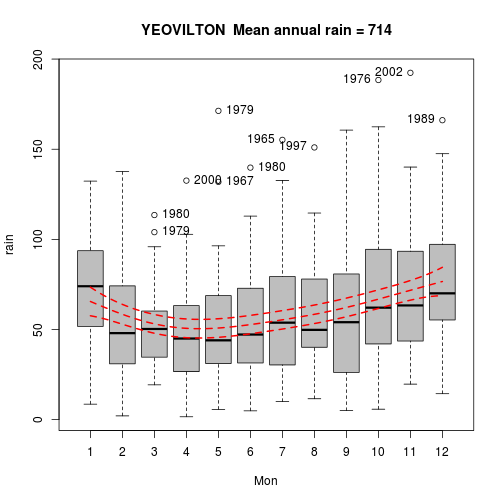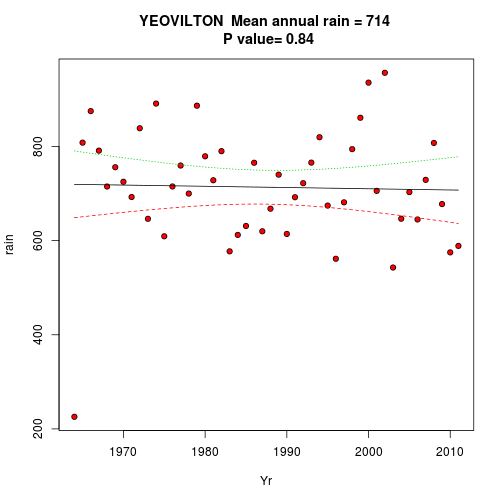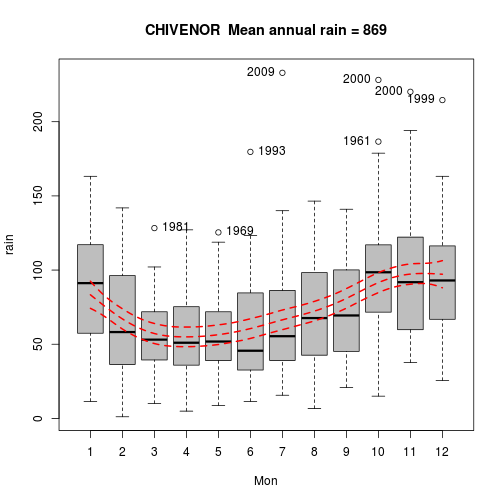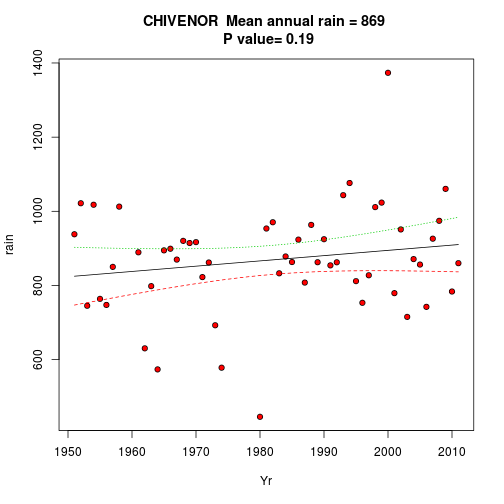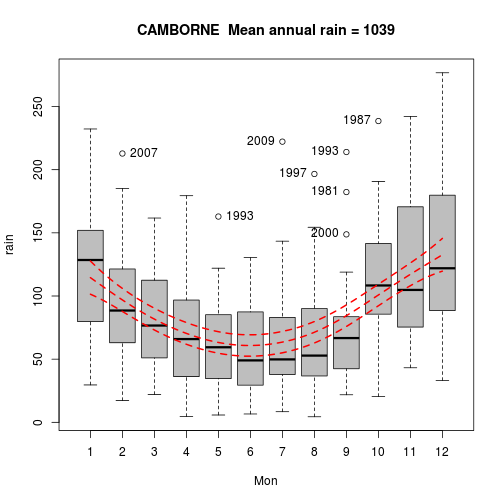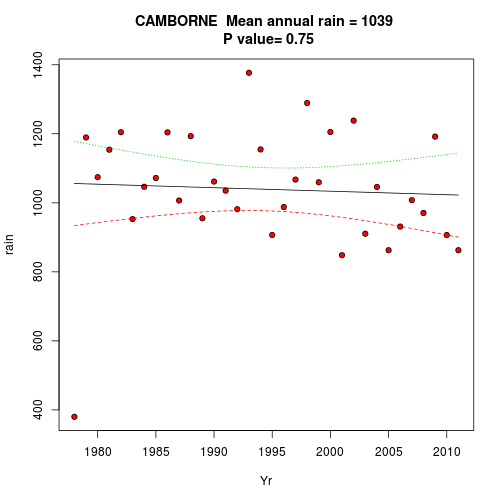``````## Warning: matrix not positive definite
``````
``````## Warning: matrix not positive definite
``````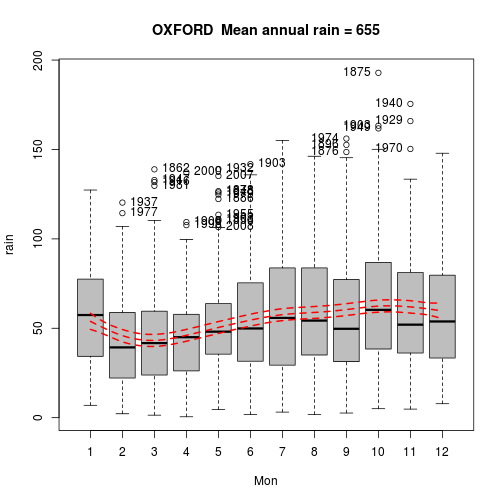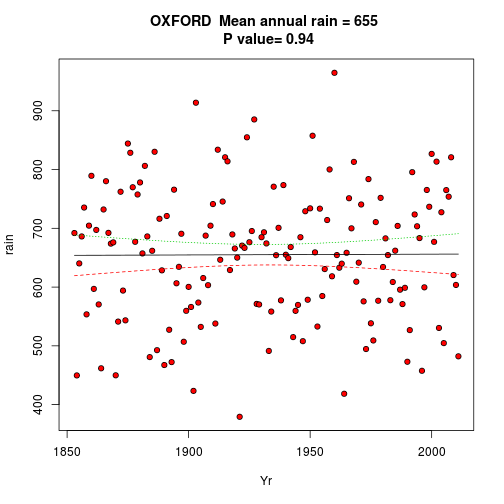``````## Warning: matrix not positive definite
``````
``````## Warning: matrix not positive definite
``````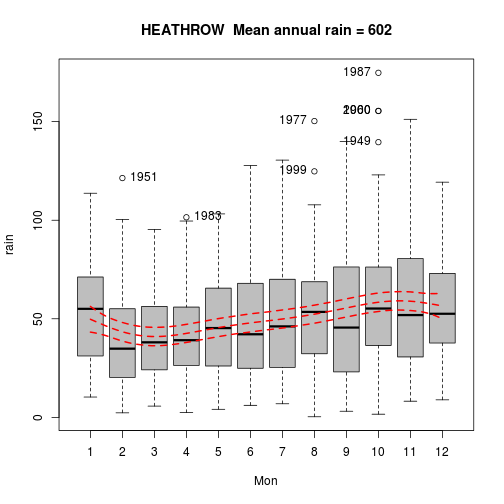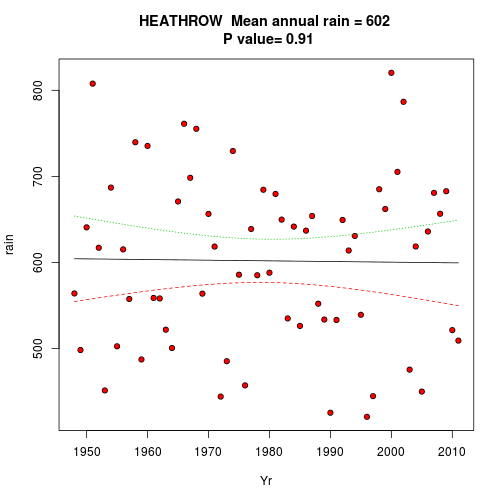``````## Warning: matrix not positive definite
``````
``````## Warning: matrix not positive definite
``````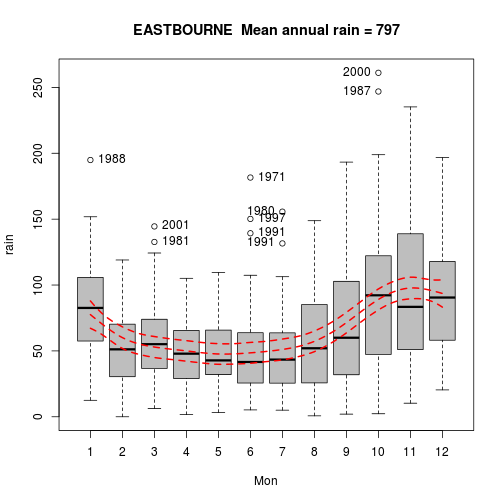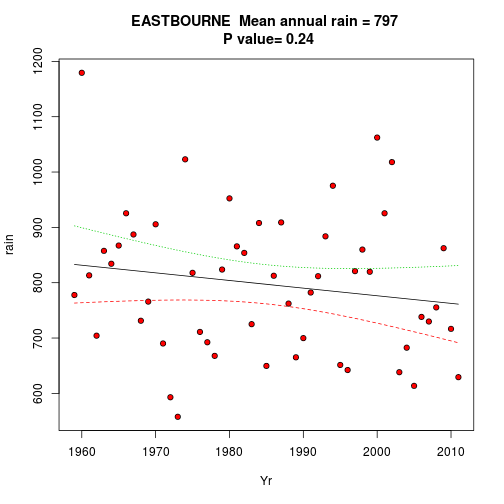``````## Warning: matrix not positive definite
``````
``````## Warning: matrix not positive definite
``````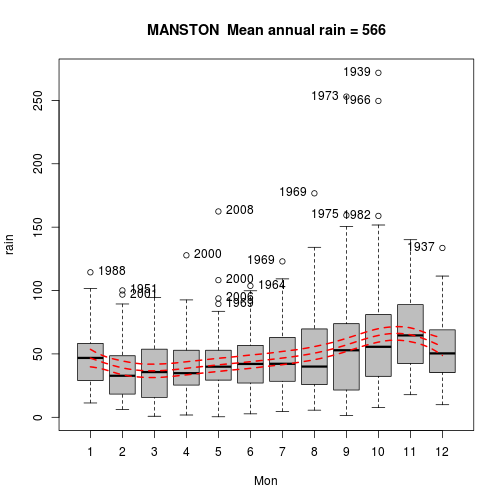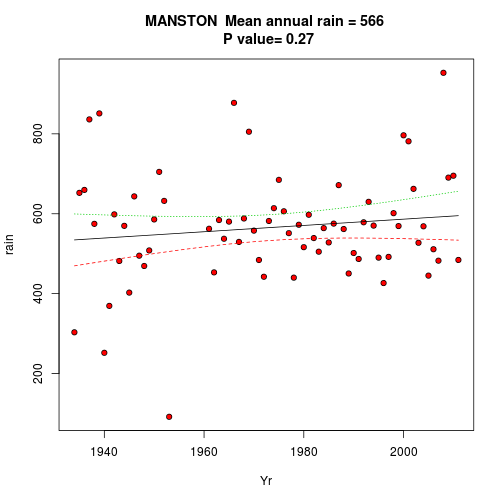``````## Warning: matrix not positive definite
``````
``````## Warning: matrix not positive definite
``````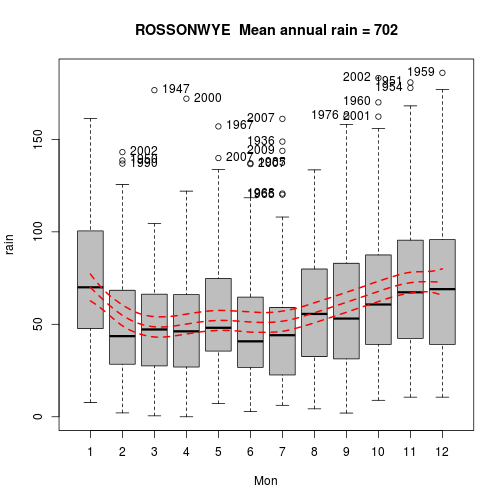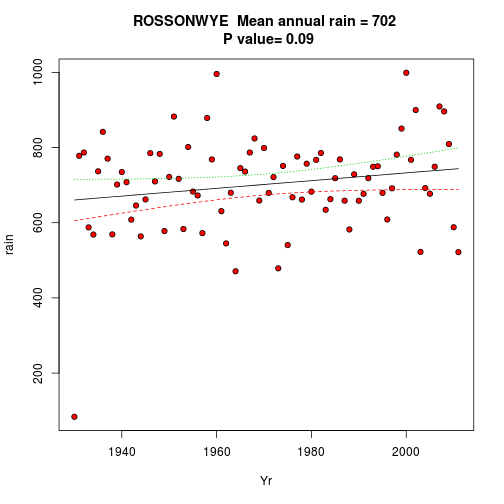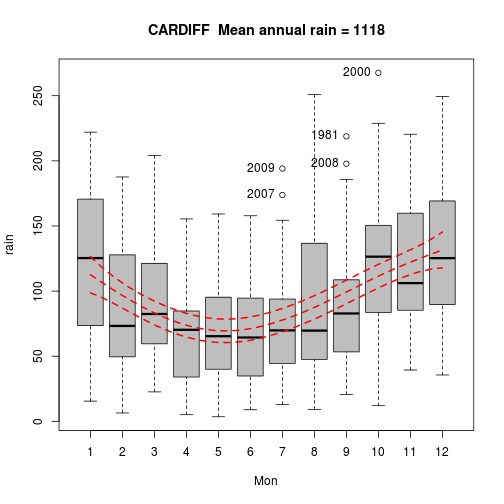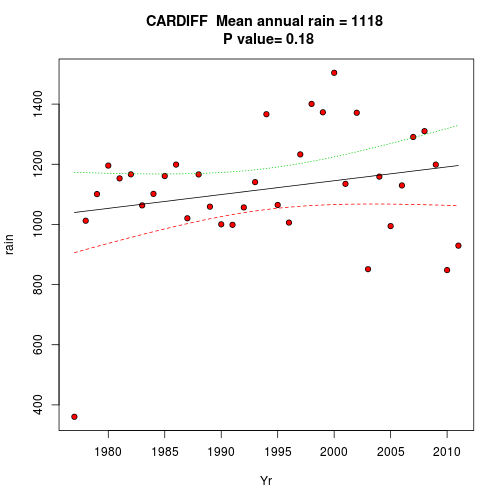``````## Warning: matrix not positive definite
``````
``````## Warning: matrix not positive definite
``````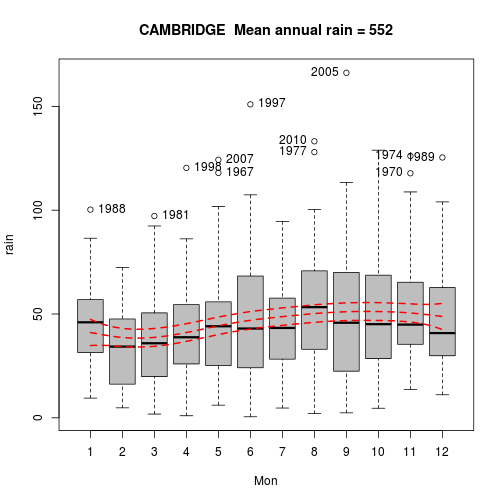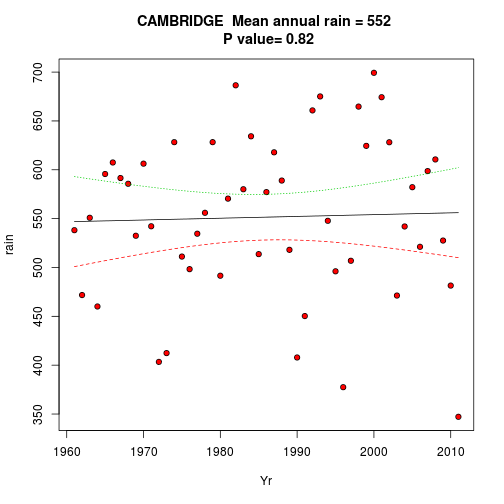``````## \$hurn
## attr(,"name")
##  "d2"
##
## \$southampton
## <environment: 0xb132b14>
## attr(,"name")
##  "d2"
##
## \$yeovilton
## <environment: 0xaa79b98>
## attr(,"name")
##  "d2"
##
## \$chivenor
## <environment: 0xb0d31cc>
## attr(,"name")
##  "d2"
##
## \$camborne
## <environment: 0xb0a0b94>
## attr(,"name")
##  "d2"
##
## \$oxford
## attr(,"name")
##  "d2"
##
## \$heathrow
## <environment: 0xb192cb0>
## attr(,"name")
##  "d2"
##
## \$eastbourne
## <environment: 0xb0c1560>
## attr(,"name")
##  "d2"
##
## \$manston
## <environment: 0xb052828>
## attr(,"name")
##  "d2"
##
## \$rossonwye
## <environment: 0xa9f98b4>
## attr(,"name")
##  "d2"
##
## \$cardiff
## <environment: 0xa974458>
## attr(,"name")
##  "d2"
##
## \$cambridge
## <environment: 0xac76c58>
## attr(,"name")
##  "d2"
##
``````

So there does not seem to be any evidence of major changes in the overall pattern of rainfall over the region.

We can do the same trick with temperatures. Some of the station data may need to be checked for completeness. Look how the most complete record (Southampton) shows an accelerating pattern of warming since 1850 that is not well modelled as a linear trend.

``````tminmodels <- function(d) {
d <- droplevels(d)
nm <- toupper(levels(d\$station))
nm <- paste(nm, " Mean annual minimum temp =", round(mean(tapply(d\$tmin,
d\$Yr, sum)), 1))

attach(d)
Boxplot(tmin ~ Mon, labels = Yr, data = d, col = "grey", main = nm)
mod <- gam(tmin ~ s(Mon), data = d)
ablines2(mod, lwd = 2, col = 2, lty = 2)
detach(d)
####################################
d1 <- melt(d, id = c("Yr", "Mon"), meas = c("tmin"))
d2 <- data.frame(cast(d1, Yr ~ variable, mean))
attach(d2)
####################################################
mod <- lm(tmin ~ Yr)
an <- anova(mod)
pval <- round(an[1, ], 4)
nm <- paste(nm, "\n", "P value=", pval)
nm <- paste(nm, "\n Increase per decade =", 10 * round(coef(mod), 3))
plot(tmin ~ Yr, pch = 21, bg = 2, main = nm)
ablines(mod)
detach(d2)
}
``````